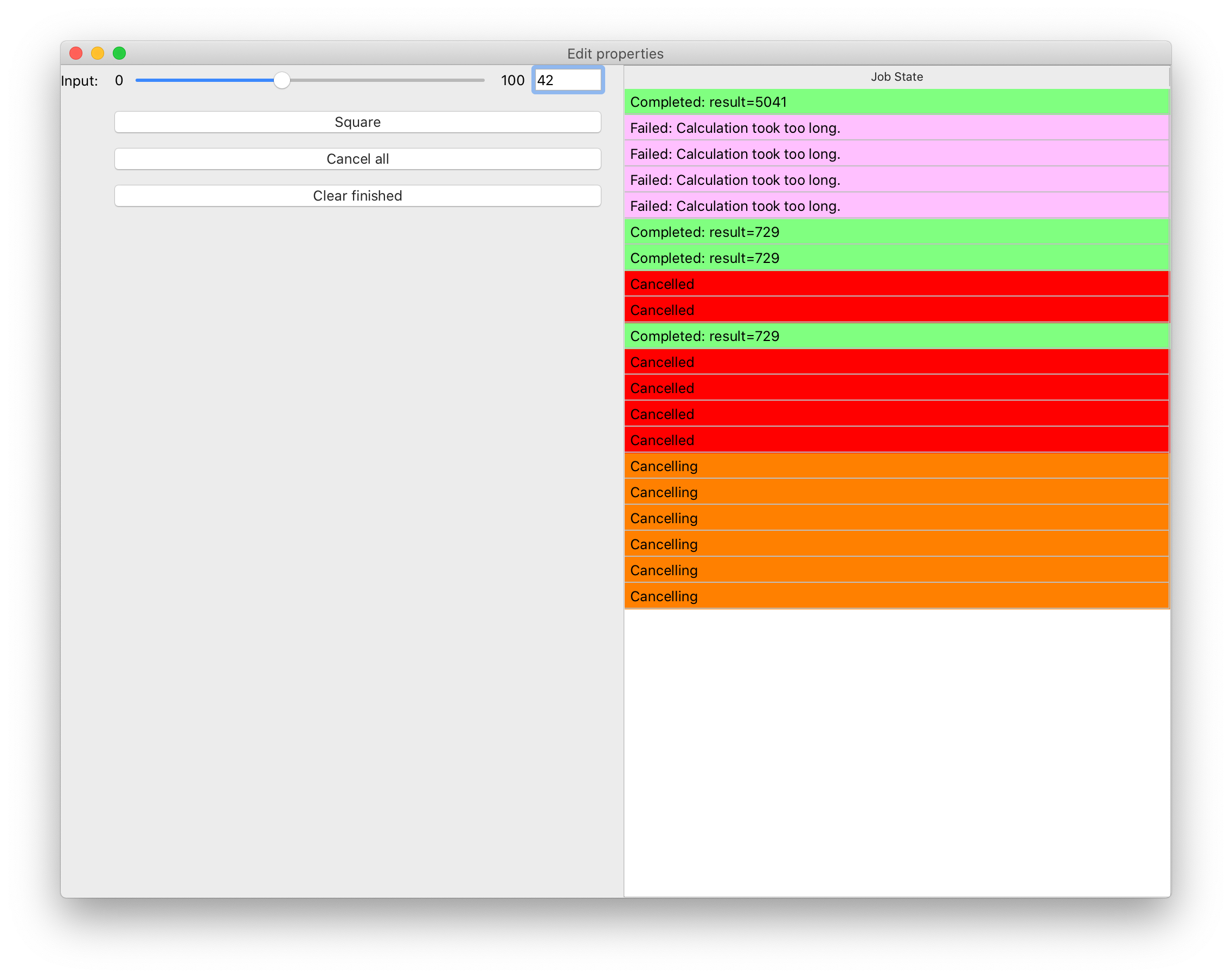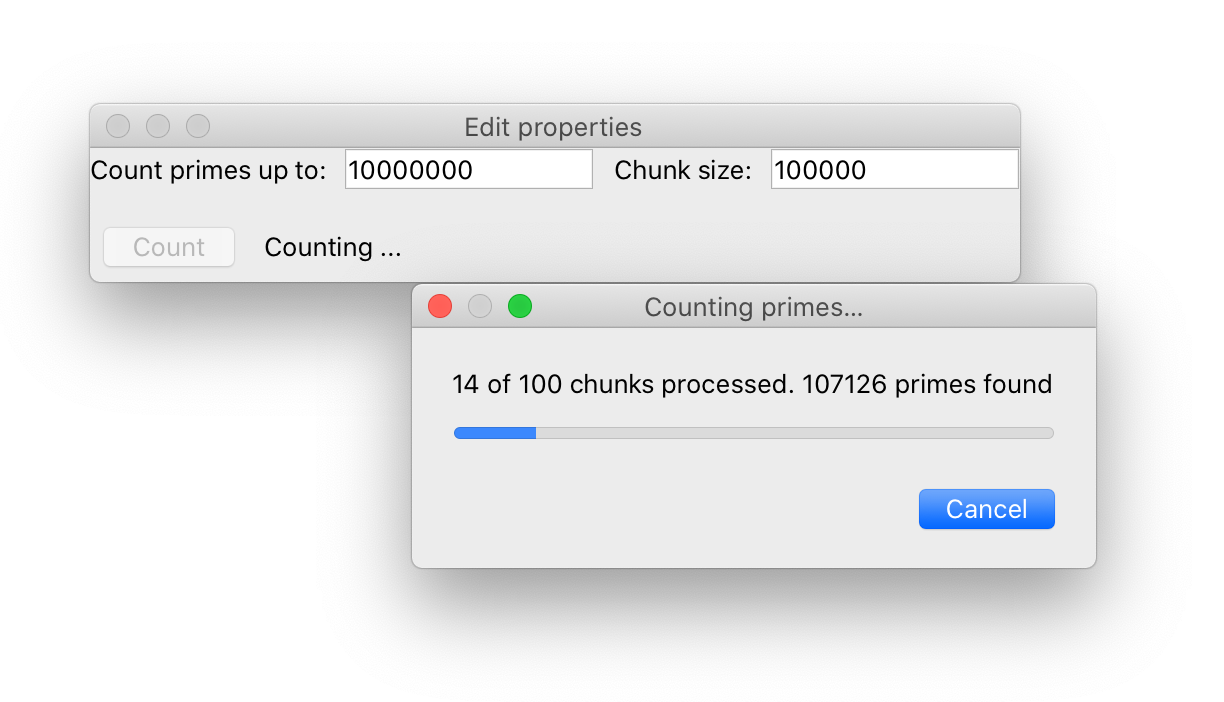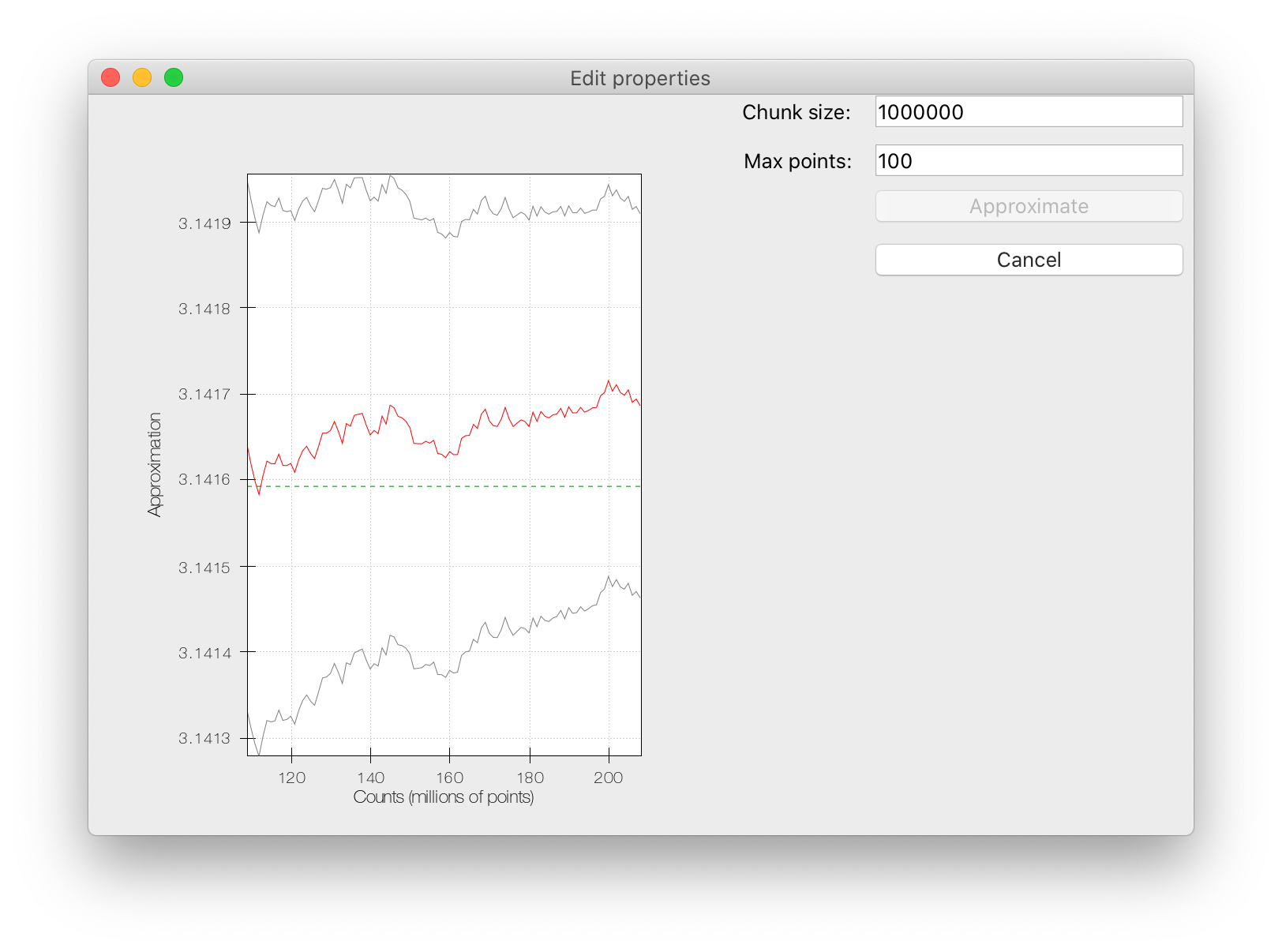# Complete examples¶

This section describes a number of complete examples, that make use of the various different background task types that Traits Futures currently supports.

## Slow squares¶This `example script` demonstrates a simple TraitsUI application that allows several background calculations to be running simultaneously, and displays the state of all previously submitted calculations in a table. Each “calculation” is a squaring operation; the calculation may fail randomly, with an increased chance of failure for larger inputs.

```import logging
import random
import time

from traits.api import (
Button,
Dict,
HasStrictTraits,
Instance,
List,
observe,
Property,
Range,
Str,
)
from traits_futures.api import (
CallFuture,
CANCELLED,
CANCELLING,
COMPLETED,
EXECUTING,
FAILED,
submit_call,
TraitsExecutor,
WAITING,
)
from traitsui.api import (
HGroup,
Item,
TabularEditor,
UItem,
VGroup,
View,
)

def slow_square(n, timeout=5.0):
"""
Compute the square of an integer, slowly and unreliably.

The input should be in the range 0-100. The larger
the input, the longer the expected time to complete the operation,
and the higher the likelihood of timeout.
"""
mean_time = (n + 5.0) / 5.0
sleep_time = random.expovariate(1.0 / mean_time)
if sleep_time > timeout:
time.sleep(timeout)
raise RuntimeError("Calculation took too long.")
else:
time.sleep(sleep_time)
return n * n

columns = [
("Job State", "state"),
]

#: Row colors for the table.
colors = Dict(
{
CANCELLED: (255, 0, 0),
CANCELLING: (255, 128, 0),
EXECUTING: (128, 128, 255),
FAILED: (255, 192, 255),
COMPLETED: (128, 255, 128),
WAITING: (255, 255, 255),
}
)

#: Text to be displayed for the state column.
state_text = Property(Str())

def _get_bg_color(self):
return self.colors[self.item.state]

def _get_state_text(self):
job = self.item
state = job.state
state_text = state.title()
if state == COMPLETED:
state_text += ": result={}".format(job.result)
elif state == FAILED:
state_text += ": {}".format(job.exception)
return state_text

class SquaringHelper(HasStrictTraits):
#: The Traits executor for the background jobs.
traits_executor = Instance(TraitsExecutor)

#: List of the submitted jobs, for display purposes.
current_futures = List(Instance(CallFuture))

#: Start a new squaring operation.
square = Button()

#: Cancel all currently executing jobs.
cancel_all = Button()

#: Clear completed jobs from the list of current jobs.
clear_finished = Button()

#: Value that we'll square.
input = Range(low=0, high=100)

@observe("square")
def _do_slow_square(self, event):
future = submit_call(self.traits_executor, slow_square, self.input)
self.current_futures.append(future)

@observe("cancel_all")
def _cancel_all_futures(self, event):
for future in self.current_futures:
future.cancel()

@observe("clear_finished")
def _clear_finished_futures(self, event):
for future in list(self.current_futures):
if future.done:
self.current_futures.remove(future)

def default_traits_view(self):
return View(
HGroup(
VGroup(
Item("input"),
UItem("square"),
UItem("cancel_all"),
UItem("clear_finished"),
),
VGroup(
UItem(
"current_futures",
editor=TabularEditor(
auto_update=True,
),
),
),
),
width=1024,
height=768,
resizable=True,
)

if __name__ == "__main__":
logging.basicConfig(
level=logging.DEBUG,
format=(
"%(asctime)s %(levelname)-8.8s [%(name)s:%(lineno)s] %(message)s"
),
)
traits_executor = TraitsExecutor()
try:
view = SquaringHelper(traits_executor=traits_executor)
view.configure_traits()
finally:
traits_executor.shutdown()
```

## Blocking call with dialog¶This `example script` demonstrates use of simple non-cancellable non-closable modal progress dialog to provide a user with visual feedback, and keep a GUI responsive, while a blocking task runs.

```"""
Example of popping up a modal progress dialog during a simple call.

Use-case: in a running GUI, we want to make a short-running computation
or service call, let's say taking a few seconds. While the computation or
call is in progress, we want to:

(a) provide some visual feedback to the user to let them know that something is
happening.
(b) free up the GUI thread so that the GUI doesn't appear to be frozen to
either the user or the operating system, avoiding system reports of the
form "Python is not responding".

The solution shown in this example is to push the computation to a background
thread using ``submit_call`` and pop up a simple non-cancellable non-closable
progress dialog while the computation is running. Since all we have is a simple
call, no actual progress is shown, but depending on the OS and Qt stylesheet
in use, Qt will often animate the progress bar.
"""

from pyface.api import Dialog
from pyface.qt import QtCore, QtGui
from traits.api import Button, HasStrictTraits, Instance, observe, Range
from traits_futures.api import CallFuture, submit_call, TraitsExecutor
from traitsui.api import Item, UItem, View

def fibonacci(n):
"""
Deliberately inefficient recursive implementation of the Fibonacci series.

Parameters
----------
n : int
Nonnegative integer - the index into the Fibonacci series.

Returns
-------
fib : int
The value of the Fibonacci series at n.
"""
return n if n < 2 else fibonacci(n - 1) + fibonacci(n - 2)

class NonClosableDialog(QtGui.QDialog):
"""
Modification of QDialog that does nothing on attempts to close.
"""

# By default, a QDialog closes when its close button is used, or when
# the "ESC" key is pressed. Both actions are routed through the dialog's
# 'reject' method, so we can override that method to prevent closing.

def reject(self):
"""
Do nothing on close.
"""

class SlowCallDialog(Dialog):
"""
Simple Pyface dialog showing a progress bar, a title, and nothing else
"""

#: The future representing the running background task.
future = Instance(CallFuture)

def _create_contents(self, parent):
# Override base class implementation to provide a simple progress bar.
progress_bar = QtGui.QProgressBar(parent)
progress_bar.setRange(0, 0)
layout = QtGui.QVBoxLayout()
parent.setLayout(layout)

def _create_control(self, parent):
# Override base class implementation in order to customize title hints,
# and in particular to remove the close button.
dlg = NonClosableDialog(
parent,
QtCore.Qt.CustomizeWindowHint | QtCore.Qt.WindowTitleHint,
)
dlg.setWindowTitle(self.title)
return dlg

@observe("future:done")
def _close_dialog_on_future_completion(self, event):
"""
Close the dialog when the background task completes.
"""
self.close()

class FibonacciCalculator(HasStrictTraits):
"""
GUI with a 'calculate' button that runs a blocking calculation.

While the blocking operation is running, a minimal progress
dialog is displayed to the user.
"""

#: The executor to submit tasks to.
traits_executor = Instance(TraitsExecutor)

#: Input for the calculation
input = Range(20, 40, 35)

#: Button to start the calculation
calculate = Button("Calculate")

@observe("calculate")
def _submit_background_call(self, event):
SlowCallDialog(
future=submit_call(self.traits_executor, fibonacci, self.input),
).open()

traits_view = View(
Item("input"),
UItem("calculate"),
)

if __name__ == "__main__":
traits_executor = TraitsExecutor()
try:
FibonacciCalculator(traits_executor=traits_executor).configure_traits()
finally:
traits_executor.shutdown()
```

## Prime counting¶This `example script` demonstrates the `ProgressFuture`, which reports progress information back to the GUI as the background computation progresses.

```"""
Example showing progress reporting from a background computation, with a
modal progress dialog.
"""
from pyface.qt import QtCore, QtGui
from pyface.ui.qt4.dialog import Dialog
from traits.api import (
Any,
Bool,
Button,
HasStrictTraits,
Instance,
Int,
observe,
Property,
Str,
)
from traits_futures.api import (
CANCELLED,
COMPLETED,
ProgressFuture,
submit_progress,
TraitsExecutor,
)
from traitsui.api import HGroup, Item, UItem, VGroup, View

class ProgressDialog(Dialog, HasStrictTraits):
"""
Dialog showing progress of the prime-counting operation.
"""

#: The future that we're listening to.
future = Instance(ProgressFuture)

#: The message to display.
message = Str()

#: The maximum number of steps.
maximum = Int(1)

#: The current step.
value = Int(0)

def cancel(self):
"""
Cancel the running future when the cancel button is pressed.
"""
self._cancel_button.setEnabled(False)
cancelled = self.future.cancel()
if cancelled:
self.message = "Cancelling\N{HORIZONTAL ELLIPSIS}"

# Private traits ##########################################################

_cancel_button = Any()

_message_control = Any()

_progress_bar = Any()

# Private methods #########################################################

def _create_contents(self, parent):
layout = QtGui.QVBoxLayout()
parent.setLayout(layout)

def _create_cancel_button(self, parent):
buttons = QtGui.QDialogButtonBox()
"Cancel", QtGui.QDialogButtonBox.RejectRole
)
self._cancel_button.setDefault(True)
buttons.rejected.connect(self.cancel, type=QtCore.Qt.QueuedConnection)
return buttons

def _create_message(self, dialog, layout):
self._message_control = QtGui.QLabel(self.message, dialog)
self._message_control.setAlignment(
QtCore.Qt.AlignTop | QtCore.Qt.AlignLeft
)
return self._message_control

def _create_progress_bar(self, dialog, layout):
self._progress_bar = QtGui.QProgressBar(dialog)
return self._progress_bar

@observe("message")
def _update_message(self, event):
message = event.new
if self._message_control is not None:
self._message_control.setText(message)

@observe("maximum")
def _update_progress_bar_maximum(self, event):
maximum = event.new
if self._progress_bar is not None:
self._progress_bar.setMaximum(maximum)

@observe("value")
def _update_progress_bar_value(self, event):
value = event.new
if self._progress_bar is not None:
self._progress_bar.setValue(value)

@observe("future:progress")
def _report_progress(self, event):
progress_info = event.new
current_step, max_steps, count_so_far = progress_info
self.maximum = max_steps
self.value = current_step
self.message = "{} of {} chunks processed. {} primes found".format(
current_step, max_steps, count_so_far
)

@observe("future:done")
def _respond_to_completion(self, event):
self.close()

def isqrt(n):
"""
Find the integer square root of a positive integer.
"""
s = n
while True:
d = n // s
if s <= d:
return s
s = (s + d) // 2

def is_prime(n):
"""
Determine whether a nonnegative integer is prime.
"""
return n >= 2 and all(n % d for d in range(2, isqrt(n) + 1))

def count_primes_less_than(n, chunk_size, progress=None):
"""
Count how many primes there are smaller than n.

Uses a deliberately inefficient algorithm.
"""
nchunks = -(-n // chunk_size)
chunks = [
(i * chunk_size, min((i + 1) * chunk_size, n)) for i in range(nchunks)
]

prime_count = 0
for chunk_index, (start, end) in enumerate(chunks):
progress((chunk_index, nchunks, prime_count))
prime_count += sum(is_prime(n) for n in range(start, end))
progress((nchunks, nchunks, prime_count))

return prime_count

class PrimeCounter(HasStrictTraits):
"""
UI to compute primes less than a given number.
"""

#: The Traits executor for the background jobs.
traits_executor = Instance(TraitsExecutor)

#: Calculation future.
future = Instance(ProgressFuture)

#: Number to count primes up to.
limit = Int(10**6)

#: Chunk size to use for the calculation.
chunk_size = Int(10**4)

#: Button to start the calculation.
count = Button()

#: Bool indicating when the count should be enabled.
count_enabled = Property(Bool, observe="future.done")

#: Result from the previous run.
result_message = Str("No previous result")

#: Limit used for most recent run.
_last_limit = Int()

@observe("count")
def _count_primes(self, event):
self._last_limit = self.limit
self.future = submit_progress(
self.traits_executor,
count_primes_less_than,
self.limit,
chunk_size=self.chunk_size,
)
self.result_message = "Counting ..."

dialog = ProgressDialog(
title="Counting primes\N{HORIZONTAL ELLIPSIS}",
future=self.future,
)
dialog.open()

def _get_count_enabled(self):
return self.future is None or self.future.done

@observe("future:done")
def _report_result(self, event):
future = event.object
if future.state == COMPLETED:
self.result_message = "There are {} primes smaller than {}".format(
future.result,
self._last_limit,
)
elif future.state == CANCELLED:
self.result_message = "Run cancelled"

def default_traits_view(self):
return View(
VGroup(
HGroup(
Item("limit", label="Count primes up to"),
Item("chunk_size"),
),
HGroup(
UItem("count", enabled_when="count_enabled"),
),
),
resizable=True,
)

if __name__ == "__main__":
traits_executor = TraitsExecutor()
try:
view = PrimeCounter(traits_executor=traits_executor)
view.configure_traits()
finally:
traits_executor.shutdown()
```

## Approximating Pi¶The final `example` uses the Chaco 2d plotting library and shows the use of the `IterationFuture`. Successive approximations to π are computed and plotted, with the plot updated live with each new value reported by the background task.

```"""
Example showing a background iteration that produces successive
approximations to pi, with resulting values being used to update
a Chaco plot.

Note: this example requires NumPy and Chaco.
"""
import numpy as np

from chaco.api import ArrayPlotData, Plot
from chaco.overlays.coordinate_line_overlay import CoordinateLineOverlay
from enable.component_editor import ComponentEditor
from traits.api import (
Bool,
Button,
Float,
HasStrictTraits,
Instance,
Int,
List,
observe,
Property,
Tuple,
)
from traits_futures.api import (
IterationFuture,
submit_iteration,
TraitsExecutor,
)
from traitsui.api import HGroup, Item, UItem, VGroup, View

def pi_iterations(chunk_size):
"""
Generate successive approximations to pi via a Monte Carlo method.

Infinite iterator producing successive approximations to pi, via the usual
Monte-Carlo method: generate random points in a square, and count the
proportion that lie in an inscribed circle.

Parameters
----------
chunk_size : int
The number of points to sample on each iteration.

Yields
------
result : tuple (int, float, float)
Tuple containing:
- the number of points generated
- the approximation to pi
- a two-sided error giving a ~95% confidence interval on the
approximation.
"""
nsamples = ninside = 0

while True:
samples = np.random.random(size=(chunk_size, 2))
nsamples += chunk_size
ninside += int(np.sum((samples * samples).sum(axis=1) <= 1.0))

# Compute approximation along with a two-sided error giving
# a ~95% confidence interval on that approximation.
#
# We use a normal approximation interval. See wikipedia for details:
# https://en.wikipedia.org/wiki/Binomial_proportion_confidence_interval
approximation = 4 * ninside / nsamples
noutside = nsamples - ninside
error = 7.839856 * np.sqrt(ninside * noutside / nsamples**3)
yield nsamples, approximation, error

class PiIterator(HasStrictTraits):
"""
View and plot of pi approximation running in the background.
"""

#: The Traits executor for the background jobs.
traits_executor = Instance(TraitsExecutor)

#: Chunk size to use for the approximations.
chunk_size = Int(1000000)

#: Calculation future.
future = Instance(IterationFuture)

#: Results arriving from the future.
results = List(Tuple(Int(), Float(), Float()))

#: Button to start the pi approximation.
approximate = Button()

#: Is the approximate button enabled?
approximate_enabled = Property(Bool(), observe="future.state")

#: Button to cancel the pi approximation.
cancel = Button()

#: Is the cancel button enabled?
cancel_enabled = Property(Bool(), observe="future.state")

#: Maximum number of points to show in the plot.
max_points = Int(100)

#: Data for the plot.
plot_data = Instance(ArrayPlotData, ())

#: The plot.
plot = Instance(Plot)

@observe("approximate")
def _calculate_pi_approximately(self, event):
self.future = submit_iteration(
self.traits_executor, pi_iterations, chunk_size=self.chunk_size
)

@observe("cancel")
def _cancel_future(self, event):
self.future.cancel()

@observe("future")
def _reset_results(self, event):
self.results = []

@observe("future:result_event")
def _record_result(self, event):
result = event.new
self.results.append(result)
self._update_plot_data()

def _get_approximate_enabled(self):
return self.future is None or self.future.done

def _get_cancel_enabled(self):
return self.future is not None and self.future.cancellable

def _update_plot_data(self):
recent_results = self.results[-self.max_points :]  # noqa: E203
# We need the reshape for the case where the results list is empty.
results = np.array(recent_results).reshape((-1, 3))
counts, approx, errors = results.T
self.plot_data.update_data(
counts=counts / 1e6,
approx=approx,
upper=approx + errors,
lower=approx - errors,
)

def _plot_default(self):
plot = Plot(self.plot_data)
self._update_plot_data()
plot.plot(("counts", "approx"), color="red")
plot.plot(("counts", "upper"), color="gray")
plot.plot(("counts", "lower"), color="gray")
plot.x_axis.title = "Counts (millions of points)"
plot.y_axis.title = "Approximation"

# Add dashed horizontal line at pi.
pi_line = CoordinateLineOverlay(
component=plot,
value_data=[np.pi],
color="green",
line_style="dash",
)
plot.underlays.append(pi_line)

# Allow extra room for the y-axis label.

return plot

def default_traits_view(self):
return View(
HGroup(
UItem("plot", editor=ComponentEditor()),
VGroup(
Item("chunk_size"),
Item("max_points"),
UItem("approximate", enabled_when="approximate_enabled"),
UItem("cancel", enabled_when="cancel_enabled"),
),
),
resizable=True,
)

if __name__ == "__main__":
traits_executor = TraitsExecutor()
try:
view = PiIterator(traits_executor=traits_executor)
view.configure_traits()
finally:
traits_executor.shutdown()
```